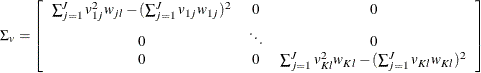### Parameter Covariance For GCE-M

Golan, Judge, and Miller (1996) give the finite approximation to the asymptotic variance matrix of the moment formulation as: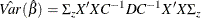where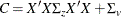and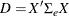Recall that in the moment formulation,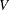is the support of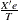, which implies that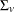is a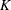-dimensional variance matrix.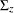andare both diagonal matrices with the form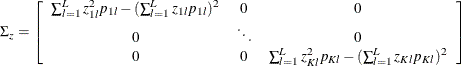and